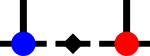SyTenHelper Scripts

# Dynamical Structure Factor Calculation

The dynamical structure factor, as measured in neutron scattering experiments, can also be calculated using DMRG. The files in this directory support the transformation from real-space/real-time correlators of the form $$\langle \hat S^+(i,t)\hat S^-(0,0) \rangle$$ or $$\langle \hat S^z(i,t)\hat S^z(0,0) \rangle$$ to momentum-space/frequency domains and the extension of data in time using linear prediction.

Specifically, we have:

• xt-kt-transform.cpp which does the Fourier transform into momentum space and potentially combines two data files (e.g. from calculating $$\langle \hat S^+(i,t)\hat S^-(0,0) \rangle$$ and $$\langle \hat S^-(i,t)\hat S^-(0,0)\rangle$$ ) into one. It expects the input data file to be a plain-text file with the first column being the time and the following columns to be real and imaginary parts of the correlators throughout the system, such as produced by e.g.
syten-apply-op -i psi-beta -o psi-beta-z64 -l 256.lat:"sz 256 @"
# evolution e^{-itH}B(0)|0⟩ of a thermal state, hence the auxiliary Hamiltonians
syten-tdvp -m 2000 -l 256.lat:"PhyHz ${Z1} * PhyHz2${Z2} * PhyHp ${P1} * PhyHp2${P2} * + + + AuxHz ${Z1} * AuxHz2${Z2} * AuxHp ${P1} * AuxHp2${P2} * + + + -1 * +" -i psi-beta-z64 -o psi-beta-z64-t -d 0.05 -T 100 -w 1e-8 --threads-tensor 4 &
# evolution e^{-itH}|0⟩ because I am lazy.
syten-tdvp -m 2000 -l 256.lat:"PhyHz ${Z1} * PhyHz2${Z2} * PhyHp ${P1} * PhyHp2${P2} * + + + AuxHz ${Z1} * AuxHz2${Z2} * AuxHp ${P1} * AuxHp2${P2} * + + + -1 * +" -i psi-beta -o psi-beta-mt -d 0.05 -T 100 -w 1e-8 --threads-tensor 1 &
for i in $(seq -f %g 0 0.05 100); do while [ ! -e psi-beta-mt_T-${i}.state ]; do
sleep 1;
done
while [ ! -e psi-beta-z64-t_T-${i}.state ]; do sleep 1; done printf "${i} " >> "${datafile}"; # -t 2 tabularises with two columns (re,im,re,im) so we can get rid # off the auxiliary-site expectation values ./syten-localexpectation -q -t 2 psi-beta-mt_T-${i}.state 256.lat sz psi-beta-z64-t_T-${i}.state -s | awk '{print$2, $3}' | tr "\n" " " >> "${datafile}";
printf "\n" >> "\${datafile}";
done
• linearPrediction.py which can be used to extend a single momentum-space slice in time using the linear prediction method. The script is rather fragile and it may be necessary to tweak some parameters, in particular self.eps.
• kt-kf-transform.cpp which does the Fourier transform into frequency space. You may also wish to adapt some parameters within the file, in particular the maximal frequency to consider.
• gen-absolute-value.sh is a helper script which reads in data files and generates new data files containing just real or imaginary parts or their absolute values suitable for plotting with gnuplot.
• apply-x.sh and apply-z.sh which run these tools for many generated result folders. You should consider these files to be more of a suggestion instead of directly usable scripts, as your requirements well may vary.

Calculation of the original correlators $$\langle \hat A(i,t)\hat B(0,0)\rangle$$ should proceed as follows:

• generate the initial state, either a ground state $$|0\rangle$$ or a thermal state using a purification.
• apply the operator $$\hat B$$ on site 0
• time-evolve the resulting state by time t. When using a purification, evolve the auxiliary system backwards to minimise entanglement.
• calculate the local expectation value of $$\langle 0|e^{it\hat H}\hat A(i)e^{-it\hat H}\hat B(0)|0\rangle$$ . You can either handle $$e^{it\hat H}$$ as a simple phase or actually evolve $$|0\rangle$$ with $$e^{-it\hat H}$$ to get $$\langle 0|e^{it\hat H}$$ for simplicity. This time-evolution will likely be extremely simple and much cheaper than the evolution $$e^{-it\hat H}\hat B(0)|0\rangle$$.